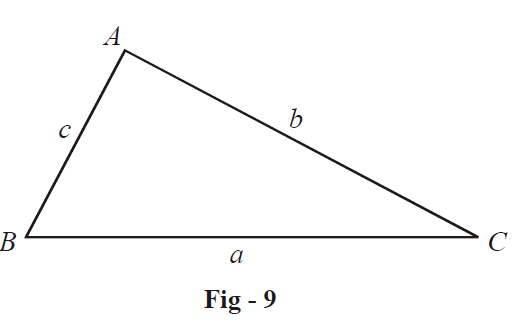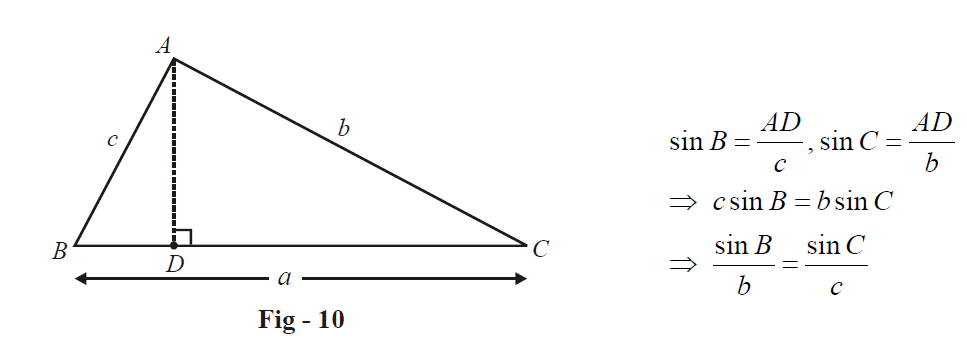# Sine Rule

Go back to  'Trigonometry'

Whenever we are discussing a triangle and its properties in general, the notation we’ll assume will correspond to the followed triangle:A triangle follows these basic properties:

(a)  $$A + B + C = \pi$$

(b) Triangle inequality:  $$a + b > c,\;b + c > a,\;c + a > b$$

These are many other simple properties of a triangle that most of you might be familiar with. In the following pages, we’ll discuss these and other properties, presenting proofs wherever necessary, and discussing applications of these properties.

### Property - 1:    Sine Rule

The sides of a triangle are proportional to the sines of the angles opposite to them:

$\frac{a}{sinA}=\frac{b}{sinB}=\frac{c}{sinC}$

We discuss the justification for the case that  $$\Delta ABC$$  is acute:The sine rule follows from extending this. Similarly, we can prove the sine rule for an obtuse angled or right angled triangle.

Note that the area of the triangle $$\Delta$$ is

$\Delta = \frac{1}{2} \times BC \times AD = \frac{1}{2}\;\;ab\;{\text{sin}}\;C = \frac{1}{2}ac\sin B$

This leads to the expression for area of a triangle:

$\boxed{\;\Delta = \frac{1}{2}ab\sin C = \frac{1}{2}\;bc\;\sin A = \frac{1}{2}ca\sin B\;}$

This is a widely used relation.